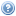# 160.111 Mathematics 1A (15 credits)

This course provides a solid mathematical foundation for further studies in mathematics, science and engineering. It consolidates basic concepts and introduces more advanced material on differentiation and numerical techniques, enabling the formation of mathematical models of real-world problems. The course blends topics from calculus with those from linear algebra and includes matrices, linear equations, vectors and geometry.

## Expected prior learning• Students should take a calculus course that includes the following Calculus Achievement Standards (or equivalent), which are Externally assessed: AS 3.5 Apply the algebra of complex numbers in solving problems AS 3.6 Apply differentiation methods in solving problems. AS 3.7 Apply integration methods in solving problems. To refresh your mathematical skills, try our basic numeracy quiz.

## OfferingsThere are no offerings currently available for this course